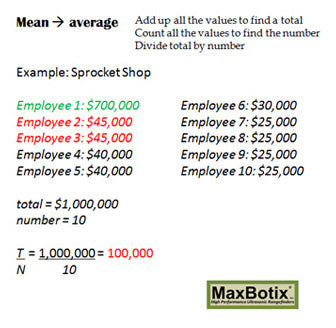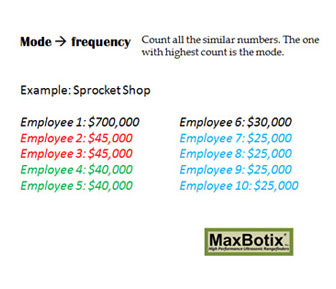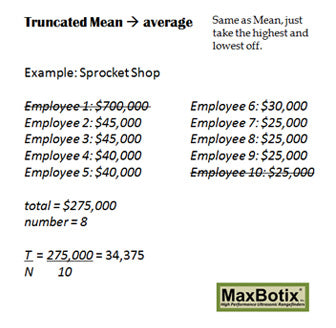# What to Know About Data Averaging

### Key Takeaways

• Data averaging can be used to smooth out unstable readings
• The mean, median, and mode are the three most common averages
• MaxBotix recommends the use of a median or mode for averaging range data

Finding an average or standard data value can be a useful way to understand the underlying trends of your data. Data averaging helps you look past random fluctuation and see the central trend of a data set. A key distinction to note early is that there are various types of averages. Each average has its own use and gives you a slightly different understanding of the central trend of a data set.

### Mean

The arithmetic mean is one of the most popular data averaging types used in the mathematical and statistical fields. The arithmetic mean is often referred to as the mean. There are other means, but for the duration of this article mean will be used to refer to an arithmetic mean. A mean is calculated by adding up all elements of the data set and dividing that value by the number of elements in the dataset. A mean adds everything up and shares it evenly amongst all elements. While the mean is easily understood and calculated, it is not without its flaws. The mean is highly influenced by outlying data.Example

Consider a fictional company, Sprocket Shop. Sprocket Shop has ten employees. If we want to understand the average annual earnings of Sprocket Shop employees we could simply calculate the mean to understand how much each employee earns if all income was shared evenly.

We add up the cumulative income to see Sprocket Shop employees earn a total of \$1,000,000 a year. We then divide that sum among the ten employees to see an average wage of \$100,000.In our example, we see that the average wage for a Sprocket Shop employee is \$100,000. Out of the ten employees, only one employee makes at least \$100,000. The second highest paid employee only earns \$45,000 which is not even half of the average wage! The mean is highly biased by very large and/or very low values. While the mean shows us how it looks if all earnings were shared evenly, it does not give us an accurate idea of the average employee’s income.

### Median

The median is another popular method of finding an average value. To find the median of a dataset, arrange all the elements from least to greatest and pick the middle value. In a case where there are two middle values, take a mean of the two middle numbers. The median is simply the middle number in your dataset. The median is the point where no more than half the values are larger than the element and no more than half the values are lower than the element. The median is considerably more resistant to outlying data points than the mean. While the median is not affected by outliers, it ignores any spread in the dataset.Let’s return to our previous example, but instead look at a median yearly income. There are two middle values: employee 6 earning \$40,000 and employee 5 earning \$30,000. The mean of these values is \$35,000, so we have an average value of \$35,000. In this case, five employees earn at least \$35,000 and five earn less than this figure.

### Mode

The final most common data averaging type is the mode. A mode is the most common reading. Whichever values show up the most is the mode. This average does look for the most popular response. A mode can be very useful in many places. It gives you the most common reading from one of our sensors, or it gives you the most popular response. For example, if you owned a shoe store you want to stock the most popular shoe sizes using the mode shoe size because the most people will request this size. The median or mean sizes will not be nearly as useful to you. However, not every dataset will have a mode. It is also possible to have more than one mode.Looking at our Sprocket Shop example we see a modal average of \$25,000 as this is the most common salary. However, no one makes less than \$25,000 so this can be a misleading figure. Especially in real-world data where a common error repeatedly occurs. A few MaxBotix Inc sensors will output a failsafe value of 0. If the failsafe gets triggered, this number can easily become your mode.

When trying to smooth observational data such as that from one of our rangefinders, a median or mode filter can often be superior to a mean filter as they are not affected by an occasional high or low reading. A mean can still be used with observational data, but we do recommend that you consider a truncated mean or a weighted mean to decrease any problems with outliers.

### Truncated Mean

When you list all of the elements of a dataset from least to greatest the outliers will fall at the two extremes. A truncated mean is calculated by taking the mean of the dataset after removing the extreme readings from the two ends. Typically, an even number of readings is removed from each end to prevent skewing the mean toward the high or low end. Truncating the mean removes the effects of outliers from this version of the mean.Again, let’s look at our fictional Sprocket Shop. If we truncate the dataset by removing the top and bottom salaries we can get a new truncated mean salary of \$34,375. We can see that this is quite a bit lower than the standard mean at \$45,000. Where only one person makes as much money as the mean, half of the employees make at least the standard mean and half make less than the truncated mean. Truncated means are a good way to help remove some of the bias of outliers in a dataset when you want to calculate a mean value.

### Which Data Averaging Type Should You Use?

Depending on what you are doing, the appropriate measure of data averaging will change. When averaging data from one of our sensors, MaxBotix Inc typically recommends a median or mode based average as one bad reading can affect the mean drastically. If you aren’t working with a sensor, are you worried about what an even distribution would look like? Then use the mean. Are you wanting to avoid high and low outliers but still get a good idea of the what the middle data points are doing? Use the median. Are you wanting to know the most popular value? Use the mode.